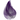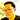# 7 Cool Tricks I Learned About Elixir Through Exercism.io

January 17, 2017## 7 Cool Tricks I Learned About Elixir Through Exercism.io

Recently, I’ve been trying to learn about functional programming language by trying out the Elixir language.

This isn’t a typical how to post so much as documenting what I’ve learned so far about Elixir’s API. A lot of it is mostly based on the code I wrote for this Elixir code kata.

### Trick 1: Rejecting a blank string in a list

In case you have to reject a blank string in a list, you can do so with code like the following. Enum.reject rejects any element for which the function result is true.

``````["H", "E", ""]
|> Enum.reject(fn(x) -> x == "" end) # reject blank string in array
# result is ["H", "E"]``````

### Trick 2: Grouping duplicate characters together

As I was working through the run-length-encoding kata, I discovered the chunkby_ method in the Enum module. Basically, this groups like characters in a list that are next to each other.

``````["H", "H", "E", "L", "L"]
|> Enum.chunk_by(fn(x) -> x end)  # group duplicate chars -> [["A", "A"], ["C", "C"]]
# result is [["H", "H"], ["E"], ["L", "L"]]``````

### Trick 3: Splitting a string via a regular expression match

If you look at the code below, it splits a string into list elements consisting of numbers and letters. Look carefully at the includecaptures_ and trim options in Code block 1 compared to Code block 2.

##### Code block 1
``````token = "3A12B"
Regex.split(~r/\d+[a-zA-Z]+/, token, [include_captures: true, trim: true])
# split string such as "3A12B" into ["3", "A", "12", "B"] without blanks``````
##### Code block 2
``````token = "3A12B"
Regex.split(~r/[a-zA-Z]+/, token)
# returns ["3", "12", ""]

Regex.split(~r/[a-zA-Z]+/, token, [include_captures: true])
#returns ["3", "A", "12", "B", ""]``````

You’ll notice both options give you some tremendous power in terms of tokenizing a string via a regular expression.

### Trick 4: Integer.parse

##### Code block 3
``````token = "3A12B"
list = Regex.split(~r/[a-zA-Z]+/, token, [include_captures: true, trim: true])
num_tuple = Integer.parse(List.first(list))
num_list = Tuple.to_list(num_tuple)
num = List.first(num_list)
letter = List.last(list)``````

### Trick 5: Tuple.to_list

Since Integer.parse(“3”) returns a tuple {3, “”}, I needed to convert it to a list. The Tuple#tolist_ method takes care of this.

`Tuple.to_list({3, ""})`

### Trick 6: Integer.to_string

To convert an integer to a string, you use the tostring_ method.

``````Integer.to_string(3)
#returns "3"``````

### Trick 7: Piping Into Anonymous Function calls

I’m showing you the complete code for context. |> (&primemessage(number, 5, &1)).()_ is piping the return value of the previous function as an argument in &1.

``````defmodule Raindrops do
@doc """
Returns a string based on raindrop factors.

- If the number contains 3 as a prime factor, output 'Pling'.
- If the number contains 5 as a prime factor, output 'Plang'.
- If the number contains 7 as a prime factor, output 'Plong'.
- If the number does not contain 3, 5, or 7 as a prime factor,
just pass the number's digits straight through.
"""

@prime_map %{
3 => "Pling",
5 => "Plang",
7 => "Plong"
}

@spec convert(pos_integer) :: String.t
def convert(number) do
r = prime_message(number, 3, "")
|> (&prime_message(number, 5, &1)).()
|> (&prime_message(number, 7, &1)).()
transform(number, r)
end

defp transform(number, r) do
if r == "" do
Integer.to_string(number)
else
r
end
end

defp prime_message(number, factor, res) do
if rem(number, factor) == 0 do
res <> @prime_map[factor]
else
res <> ""
end
end

end``````

### Summary